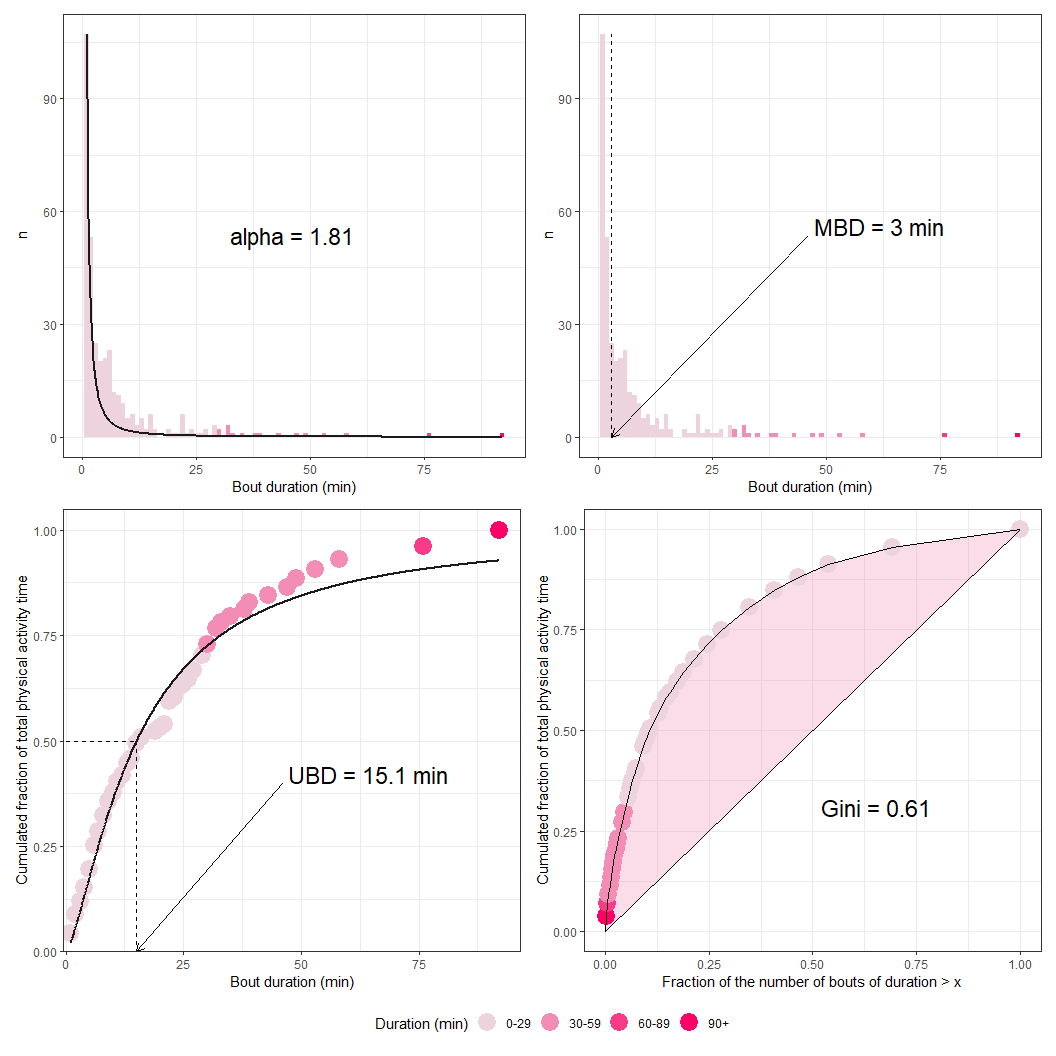# activAnalyzer

The activAnalyzer package was primarily built for working through a Shiny app. The procedure for using the app is explained in the related user’s guide. The functions used in this app can also be used to analyze data outside the app, as shown below.

library(activAnalyzer)
library(magrittr)
library(ggplot2)
library(patchwork)
library(dplyr)

## Getting file

file <- system.file("extdata", "acc.agd", package = "activAnalyzer")

## Preparing dataset

mydata <- prepare_dataset(data = file)

## Getting nonwear/wear time marks

mydata_with_wear_marks <-
mydata %>%
mark_wear_time(
to_epoch = 60,
cts = "vm",
frame = 90,
allowanceFrame = 2,
streamFrame = 30
)
#> frame is 90
#> streamFrame is 30
#> allowanceFrame is 2

## Viewing data with nonwear/wear time marks

plot_data(data = mydata_with_wear_marks, metric = "vm")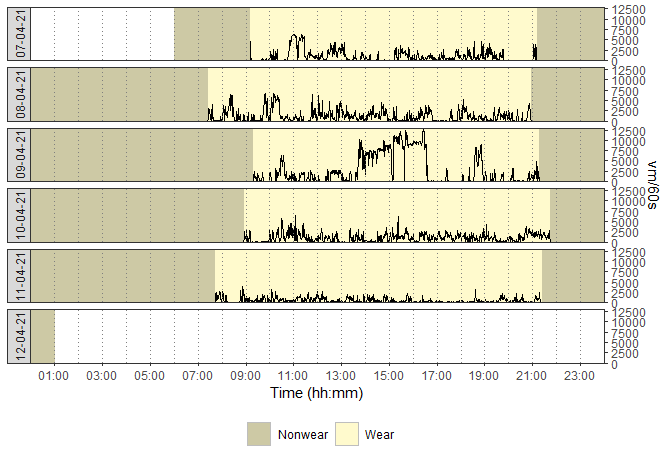## Viewing data with nonwear/wear time marks and with a zoom in on the figure

plot_data(
data = mydata_with_wear_marks,
metric = "vm",
zoom_from = "16:00:00",
zoom_to = "18:00:00"
)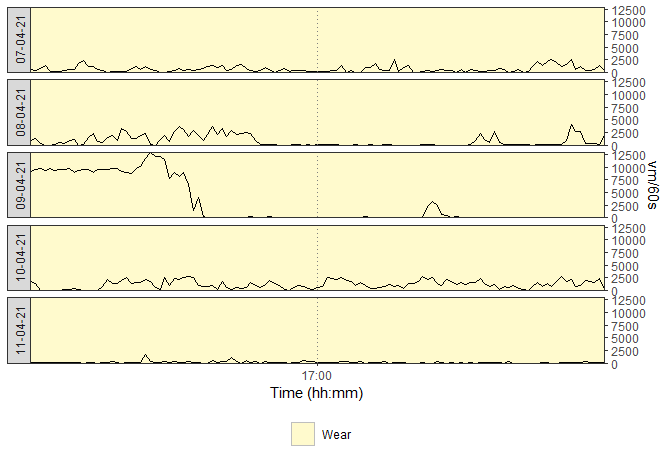## Getting activity intensity marks

mydata_with_intensity_marks <-
mark_intensity(
data = mydata_with_wear_marks,
col_axis = "vm",
equation = "Sasaki et al. (2011) [Adults]",
sed_cutpoint = 200,
mpa_cutpoint = 2690,
vpa_cutpoint = 6167,
age = 32,
weight = 67,
sex = "male"
)

## Viewing data with activity intensity marks

plot_data_with_intensity(
mydata_with_intensity_marks,
metric = "vm",
valid_wear_time_start = "00:00:00",
valid_wear_time_end = "23:59:59"
)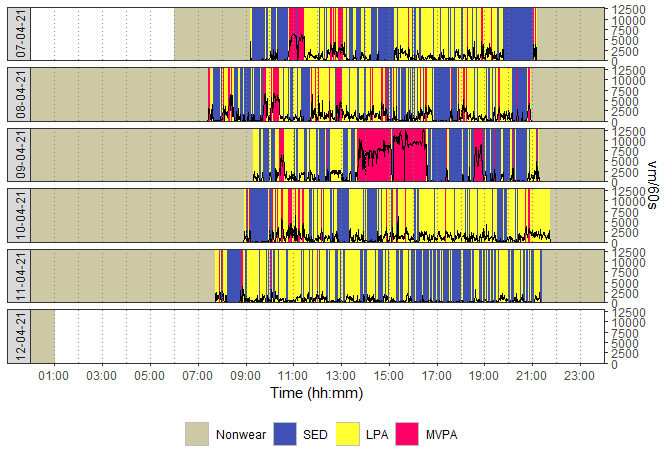## Viewing data with activity intensity marks and grey bands to highlight the daily period considered for analysis

plot_data_with_intensity(
mydata_with_intensity_marks,
metric = "vm",
valid_wear_time_start = "07:00:00",
valid_wear_time_end = "22:00:00"
)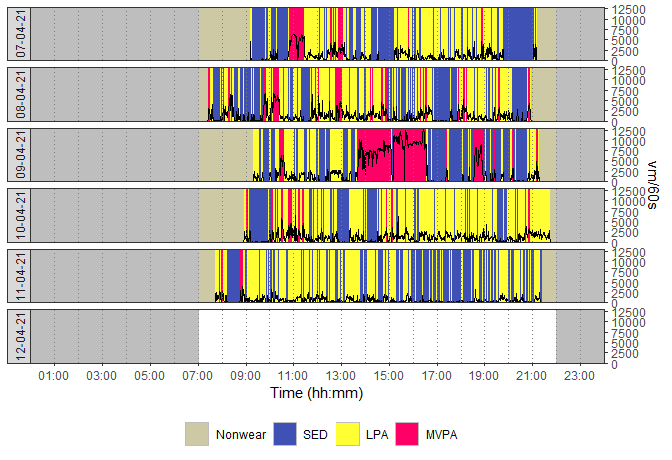## Viewing data with activity intensity marks and a zoom in on the figure

plot_data_with_intensity(
mydata_with_intensity_marks,
metric = "vm",
zoom_from = "13:00:00",
zoom_to = "16:30:00"
)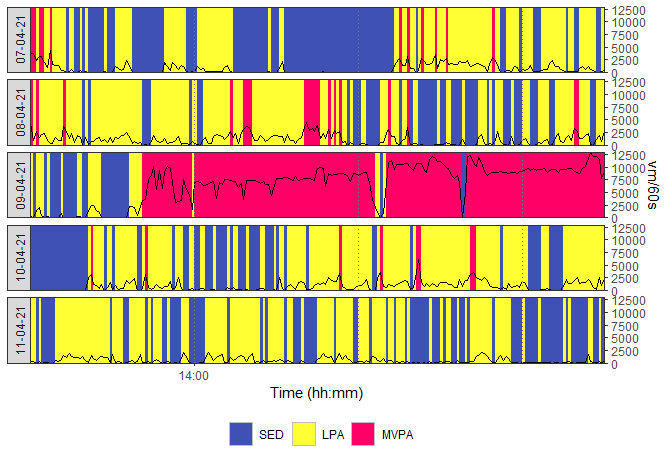## Getting activity metrics by day

results_by_day <-
mydata_with_intensity_marks %>%
recap_by_day(
age = 32,
weight = 67,
sex = "male",
valid_wear_time_start = "07:00:00",
valid_wear_time_end = "22:00:00",
start_first_bin = 0,
start_last_bin = 10000,
bin_width = 500
)

## Computing means of the metrics using the valid days

mean_results <-
results_by_day$df_all_metrics %>% average_results(minimum_wear_time = 10, fun = "mean") ## Computing medians of the metrics using the valid days median_results <- results_by_day$df_all_metrics  %>%
average_results(minimum_wear_time = 10, fun = "median")

## Showing activity volume metrics

results_by_daydf_all_metrics %>% dplyr::select(date:total_steps) %>% reactable::reactable(striped = TRUE, defaultColDef = reactable::colDef(align = "center", minWidth = 180)) ### Results by day: Graphical view create_fig_res_by_day( results_by_daydf_all_metrics,
minimum_wear_time_for_analysis = 10,
start_day_analysis = "00:00:00",
end_day_analysis = "23:59:00",
metrics = "volume",
epoch_label = "60s"
) + theme(plot.margin = margin(1, 1, 1, 1, "cm"))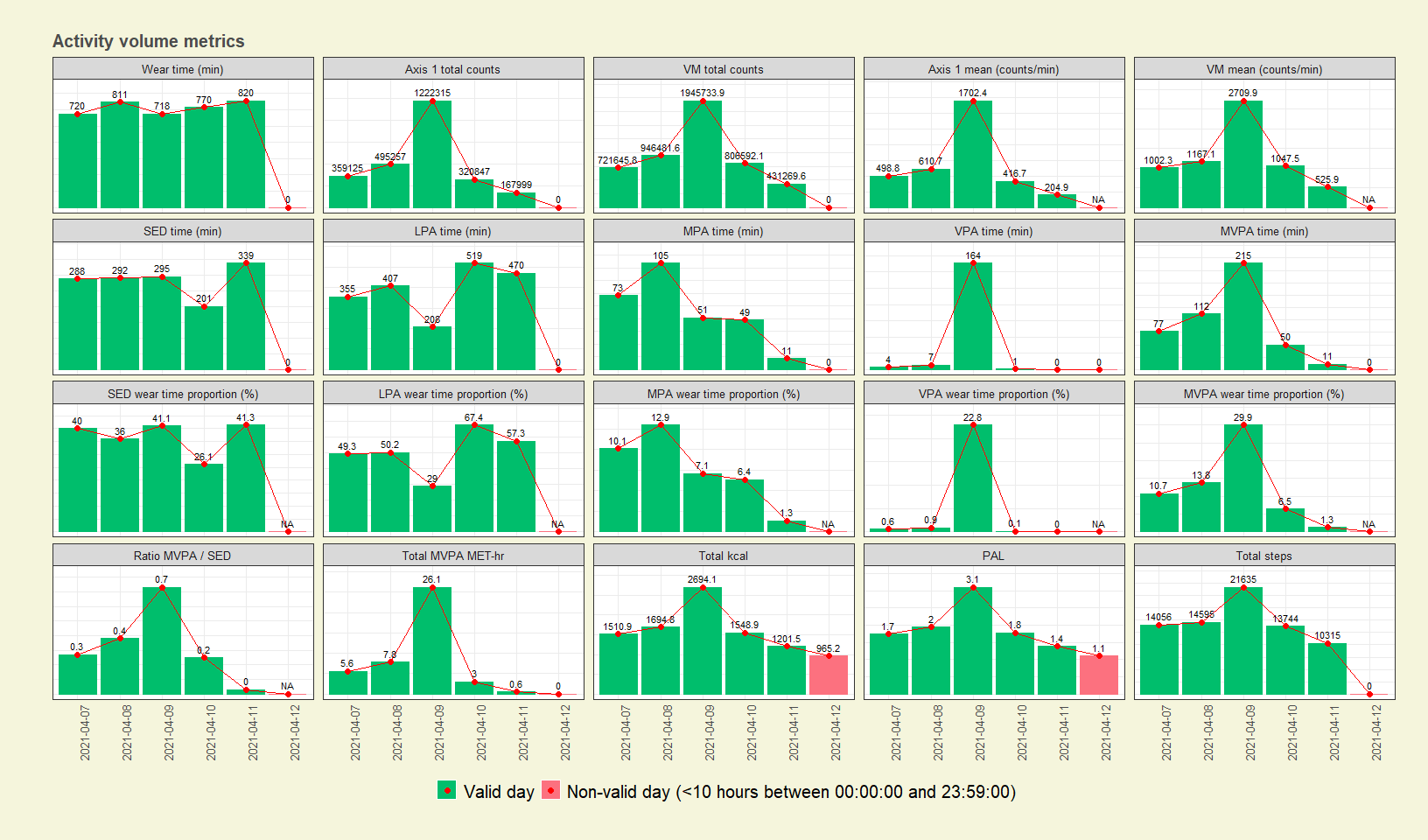### Means | Medians computed using valid days

create_flextable_summary(
results_summary_means = mean_results,
results_summary_medians = median_results,
metrics = "volume",
epoch_label = "60s"
)

Metric

Daily mean | median

Number of valid days

5

Wear time (min)

767.8 (12:47:48) | 770.0 (12:50:00)

Axis 1 total counts

513108.6 | 359125.0

VM total counts

970344.6 | 806592.1

Axis 1 mean (counts/min)

686.7 | 498.8

VM mean (counts/min)

1290.6 | 1047.5

SED time (min)

283.0 (04:43:00) | 292.0 (04:52:00)

LPA time (min)

391.8 (06:31:48) | 407.0 (06:47:00)

MPA time (min)

57.8 (00:57:48) | 51.0 (00:51:00)

VPA time (min)

35.2 (00:35:12) | 4.0 (00:04:00)

MVPA time (min)

93.0 (01:33:00) | 77.0 (01:17:00)

SED wear time proportion (%)

36.9 | 40.0

LPA wear time proportion (%)

50.6 | 50.2

MPA wear time proportion (%)

7.6 | 7.1

VPA wear time proportion (%)

4.9 | 0.6

MVPA wear time proportion (%)

12.4 | 10.7

Ratio MVPA / SED

0.33 | 0.27

Total MVPA MET-hr

8.63 | 5.56

Total kcal

1730.04 | 1548.93

PAL

1.99 | 1.78

Total steps

14869 | 14056

### Comparisons with norms and recommendations

# PAL
g_pal <- create_fig_pal(score = mean_results[["pal"]], "en") + theme(plot.margin = margin(2, 1, 0.5, 1, "cm"))

# Steps
g_steps <- create_fig_steps(score = mean_results[["total_steps"]], "en") + theme(plot.margin = margin(0, 1, 0.5, 1, "cm"))

# MVPA
g_mvpa <- create_fig_mvpa(score = mean_results[["minutes_MVPA"]], "en") + theme(plot.margin = margin(0, 1, 0, 1, "cm"))

# SED
g_sed <- create_fig_sed(score = mean_results[["minutes_SED"]], "en") + theme(plot.margin = margin(0, 1, 0, 1, "cm"))

# MVPA/SED ratio
g_ratio <- create_fig_ratio_mvpa_sed(score = mean_results[["ratio_mvpa_sed"]], "en") + theme(plot.margin = margin(0, 1, 1, 1, "cm"))

# Whole figure
g_pal / g_steps / (g_mvpa | g_sed | g_ratio) +
plot_layout(heights = c(0.8, 0.7, 1.5)) & theme(legend.justification = "center")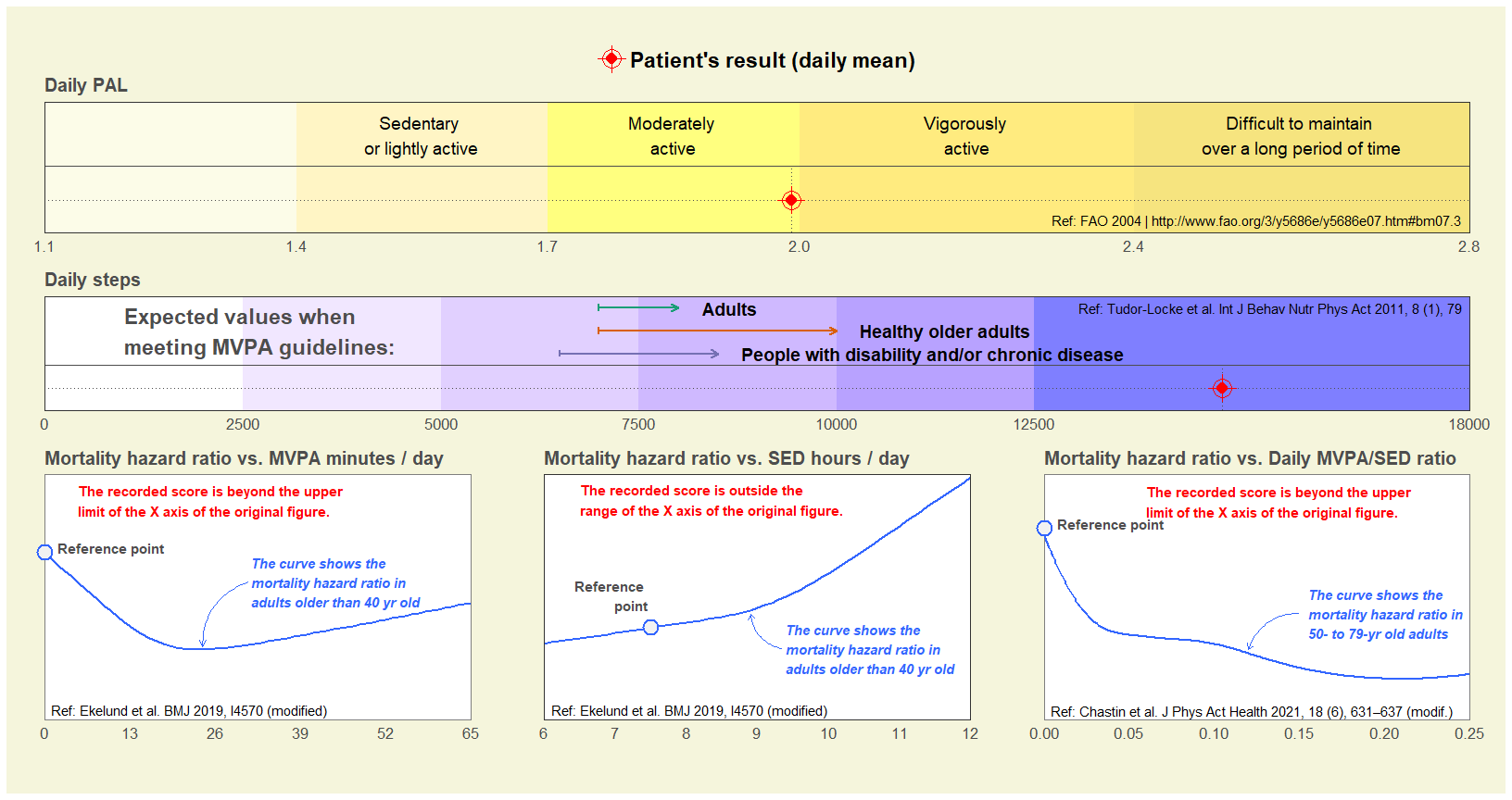## Showing step accumulation metrics

results_by_daydf_all_metrics %>% dplyr::select(date, max_steps_60min:peak_steps_1min) %>% reactable::reactable(striped = TRUE, defaultColDef = reactable::colDef(align = "center", minWidth = 180)) ### Results by day: Graphical view create_fig_res_by_day( results_by_daydf_all_metrics,
minimum_wear_time_for_analysis = 10,
start_day_analysis = "00:00:00",
end_day_analysis = "23:59:00",
metrics = "step_acc",
epoch_label = "60s"
) + theme(plot.margin = margin(1, 1, 1, 1, "cm"))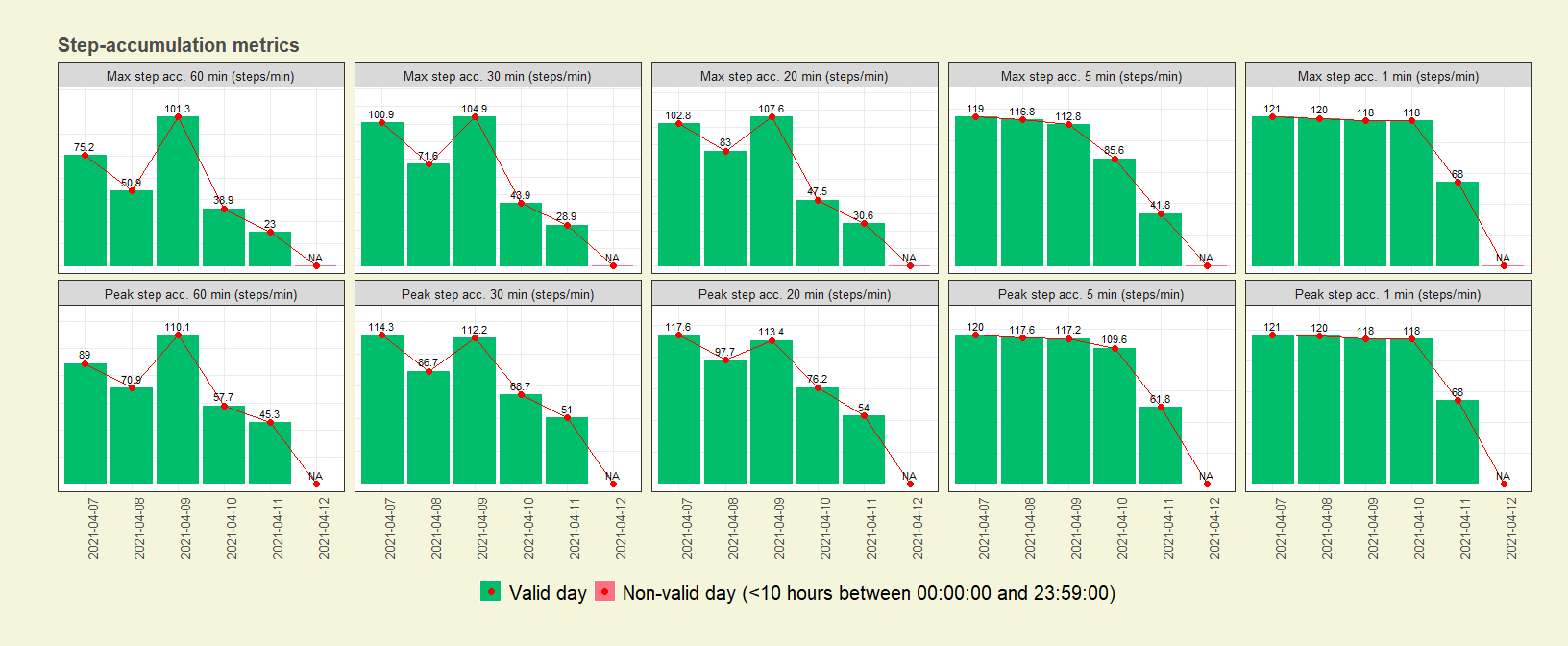### Means | Medians computed using valid days

create_flextable_summary(
results_summary_means = mean_results,
results_summary_medians = median_results,
metrics = "step_acc",
epoch_label = "60s"
)

Metric

Daily mean | median

Number of valid days

5

Max step acc. 60 min (steps/min)

57.85 | 50.90

Max step acc. 30 min (steps/min)

70.05 | 71.63

Max step acc. 20 min (steps/min)

74.29 | 83.05

Max step acc. 5 min (steps/min)

95.20 | 112.80

Max step acc. 1 min (steps/min)

109.00 | 118.00

Peak step acc. 60 min (steps/min)

74.60 | 70.88

Peak step acc. 30 min (steps/min)

86.60 | 86.73

Peak step acc. 20 min (steps/min)

91.75 | 97.70

Peak step acc. 5 min (steps/min)

105.24 | 117.20

Peak step acc. 1 min (steps/min)

109.00 | 118.00

## Showing intensity gradient and MX metrics

### Distribution of time spent in intensity bins

  results_by_day$p_band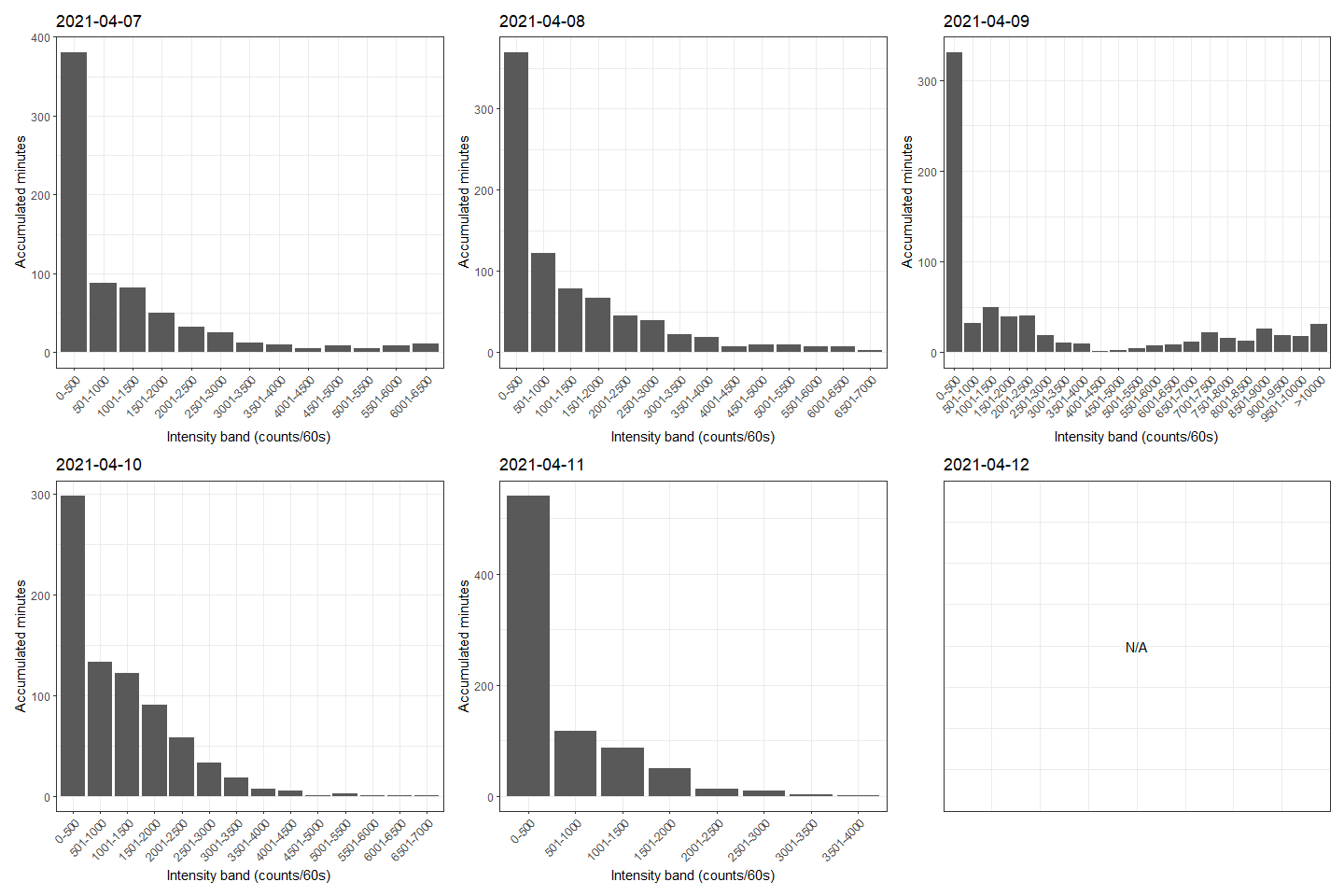results_by_day$p_log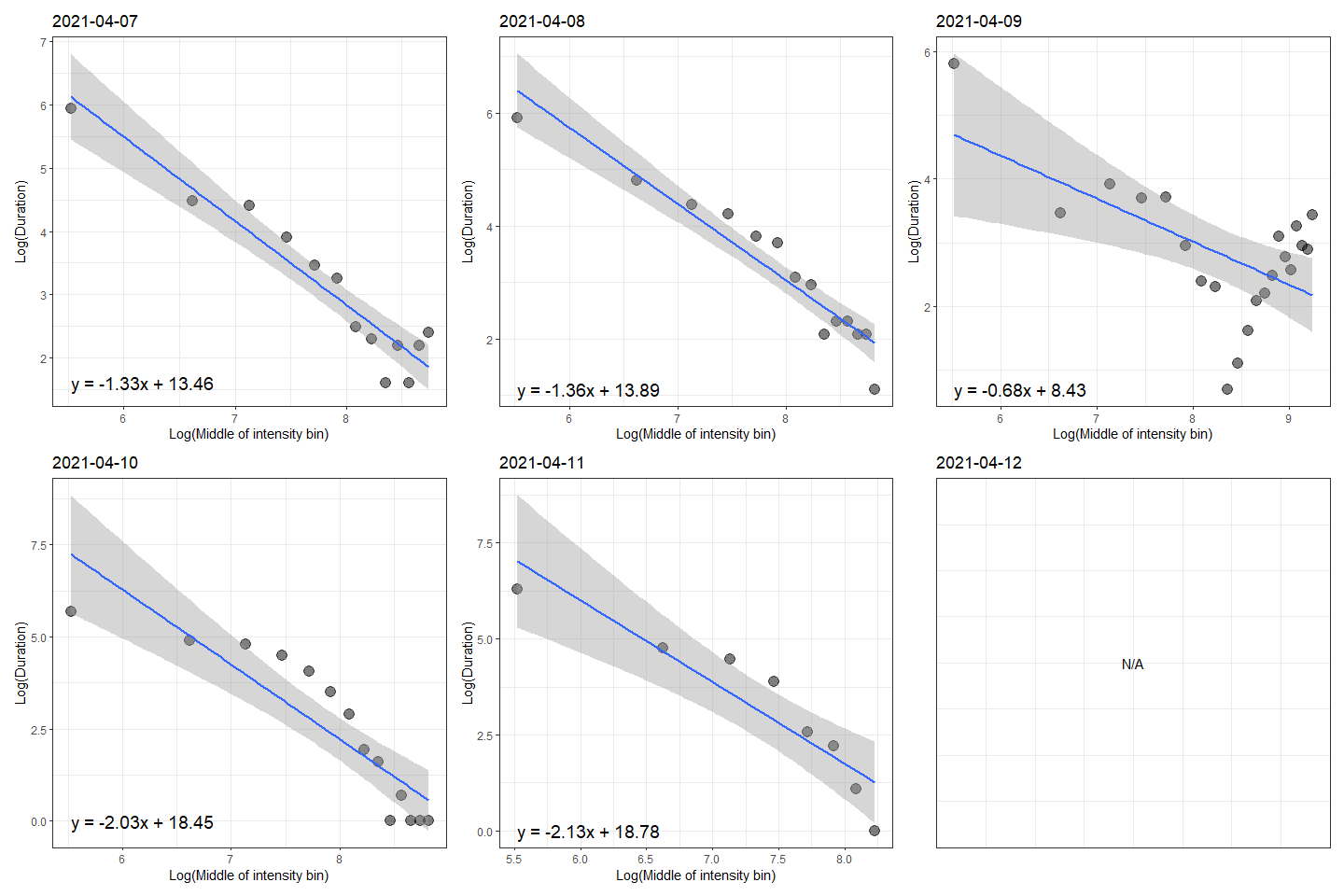results_by_daydf_all_metrics %>% dplyr::select(date, ig:M5) %>% reactable::reactable(striped = TRUE, defaultColDef = reactable::colDef(align = "center", minWidth = 180)) ### Results by day: Graphical view create_fig_res_by_day( results_by_daydf_all_metrics,
minimum_wear_time_for_analysis = 10,
start_day_analysis = "00:00:00",
end_day_analysis = "23:59:00",
metrics = "step_acc",
epoch_label = "60s"
) + theme(plot.margin = margin(1, 1, 1, 1, "cm"))### Means | Medians computed using valid days

create_flextable_summary(
results_summary_means = mean_results,
results_summary_medians = median_results,
metrics = "int_distri",
epoch_label = "60s"
)

Metric

Daily mean | median

Number of valid days

5

-1.51 | -1.36

M1/3 (counts/60s)

1983.5 | 1600.9

M120 (counts/60s)

4330.7 | 3585.2

M60 (counts/60s)

5123.3 | 4706.1

M30 (counts/60s)

5830.0 | 5678.7

M15 (counts/60s)

6311.4 | 6073.2

M5 (counts/60s)

6824.5 | 6229.5

### Radar view based on the means of valid days

create_fig_mx_summary(
data = mean_results,
labels = NULL,
mpa_cutpoint = 2690,
vpa_cutpoint = 6167
)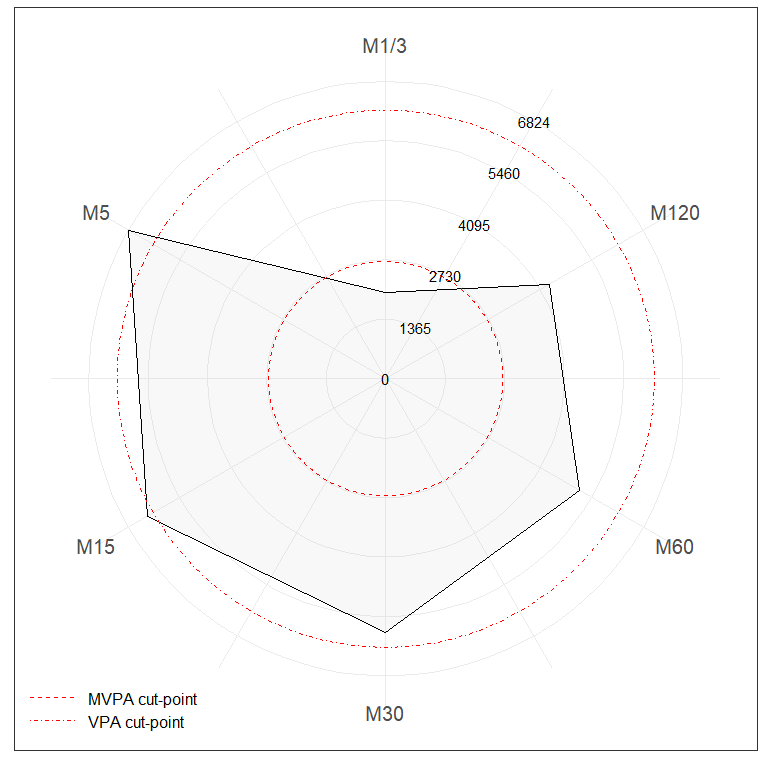## Showing sedentary behaviour accumulation metrics based on valid days

### Getting results

accum_metrics_sed <-
compute_accumulation_metrics(
data = mydata_with_intensity_marks,
behaviour = "sed",
dates = c("2021-04-07", "2021-04-08", "2021-04-09", "2021-04-10", "2021-04-11")
)

accum_metrics_sed$p_breaks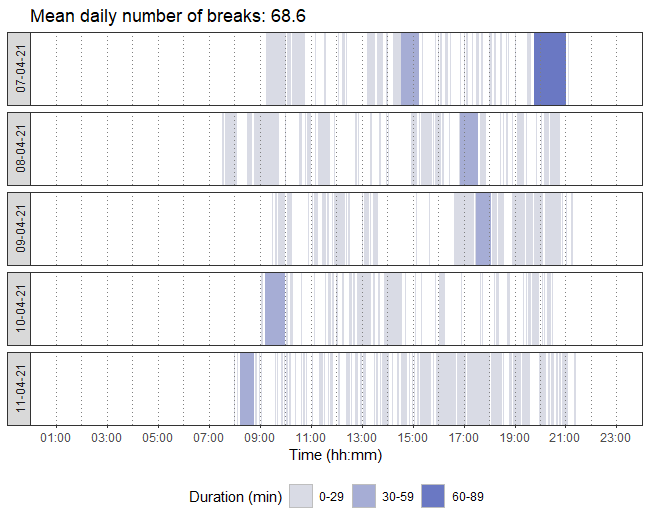### Alpha coefficient, median bout duration (MBD), usual bout duration (UBD), and Gini index p1 <- accum_metrics_sed$p_alpha  + guides(color = "none", fill = "none")
p2 <- accum_metrics_sed$p_MBD + guides(color = "none", fill = "none") p3 <- accum_metrics_sed$p_UBD
p4 <- accum_metrics_sed$p_gini (p1 | p2) / (p3 | p4) + plot_layout(guides = "collect") & theme(legend.position = 'bottom')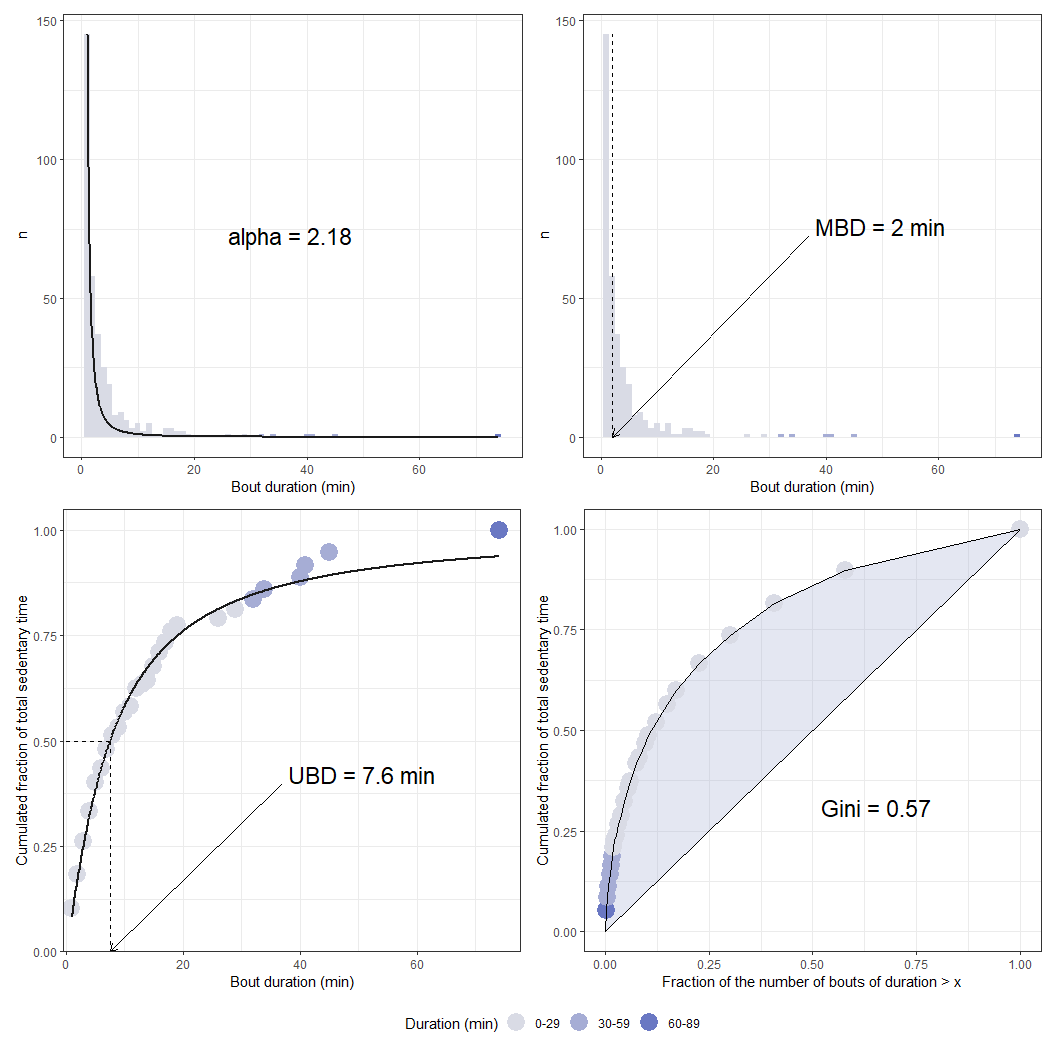## Showing physical activity accumulation metrics based on valid days ### Getting results accum_metrics_pa <- compute_accumulation_metrics( mydata_with_intensity_marks, behaviour = "pa", dates = c("2021-04-07", "2021-04-08", "2021-04-09", "2021-04-10", "2021-04-11") ) ### Temporal distribution of physical activity bouts accum_metrics_pa$p_breaks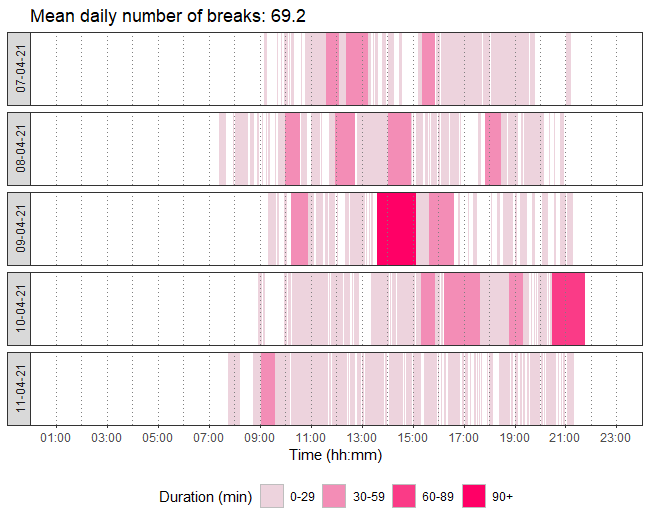### Alpha coefficient, median bout duration (MBD), usual bout duration (UBD), and Gini index

p1 <- accum_metrics_pa$p_alpha + guides(color = "none", fill = "none") p2 <- accum_metrics_pa$p_MBD    + guides(color = "none", fill = "none")
p3 <- accum_metrics_pa$p_UBD p4 <- accum_metrics_pa$p_gini

(p1 | p2) / (p3 | p4) + plot_layout(guides = "collect") & theme(legend.position = 'bottom')